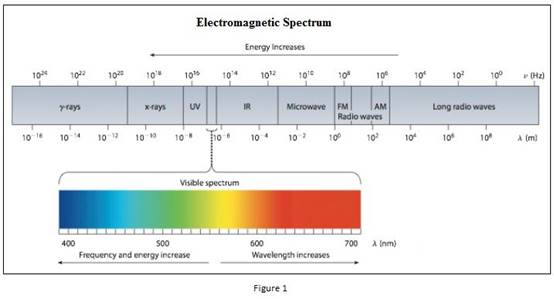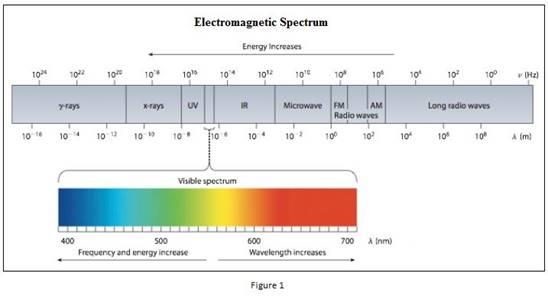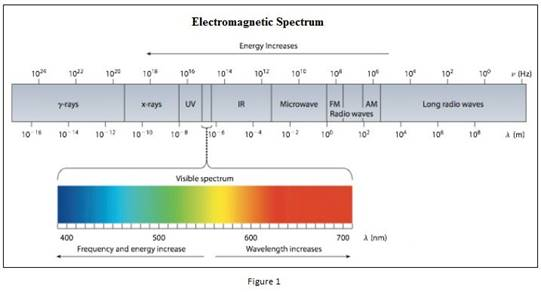Chapter 6, Problem 55GQ

Chapter
Section
Textbook Problem

An advertising sign gives off red light and green light. (a) Which light has higher-energy photons? (b) One of the colors has a wavelength of 680 nm, and the other has a wavelength of 500 nm. Which color has which wavelength? (c) Which light has the higher frequency?

(a)

Interpretation Introduction

Interpretation: Among green light and red light, the colour having the higher energy photons should be identified.

Concept introduction:

• Electromagnetic radiations are a type of energy surrounding us. They are of different types like radio waves, IR, UV, X-ray etc.• The red light and green light lies in the visible light where it is between 400nm and 700nm.
• Planck’s equation,

E==hcλwhere, E=energyh=Planck'sconstantν=frequency

The energy increases as the wavelength of the light decrease. Also the energy increases as the frequency of the light increases.

• The frequency of the light is inversely proportional to its wavelength.

ν=cλwhere, c=speedoflightν=frequencyλ=wavelength

Explanation

E=hcλ so the energy increases as the wavelength of the light decrease

(b)

Interpretation Introduction

Interpretation: Among green light and red light, the colour having 500nm and 680nm wavelength should be identified.

Concept introduction:

• Electromagnetic radiations are a type of energy surrounding us. They are of different types like radio waves, IR, UV, X-ray etc.• The red light and green light lies in the visible light where it is between 400nm and 700nm.

(c)

Interpretation Introduction

Interpretation: Among green light and red light, the colour having higher frequency should be identified.

Concept introduction:

• Electromagnetic radiations are a type of energy surrounding us. They are of different types like radio waves, IR, UV, X-ray etc.• The red light and green light lies in the visible light where it is between 400nm and 700nm.
• The frequency of the light is inversely proportional to its wavelength.

ν=cλwhere, c=speedoflightν=frequencyλ=wavelength

Still sussing out bartleby?

Check out a sample textbook solution.

See a sample solution

The Solution to Your Study Problems

Bartleby provides explanations to thousands of textbook problems written by our experts, many with advanced degrees!

Get Started

What is a peptide bond, and what type of reaction forms it?

Biology: The Dynamic Science (MindTap Course List)

What types of forces give rise to quaternary structure?

Chemistry for Today: General, Organic, and Biochemistry

Whats a monsoon? Do we experience monsoons in the continental United States?

Oceanography: An Invitation To Marine Science, Loose-leaf Versin